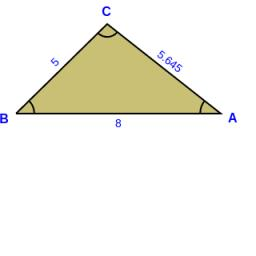# Construct 4129

Construct a triangle ABC, given the lengths of the sides
c = 8 cm, a = 5 cm and length length Vc = 3.5 cm.
Perform an analysis, write down the design procedure, perform it, and determine the number of solutions.

b1 =  12.088 cm
b2 =  5.645 cm

### Step-by-step explanation:

Try calculation via our triangle calculator.Did you find an error or inaccuracy? Feel free to write us. Thank you!

Tips for related online calculators
Do you want to convert length units?

#### Grade of the word problem:

We encourage you to watch this tutorial video on this math problem: